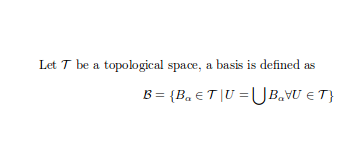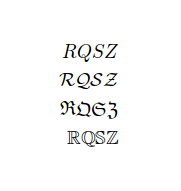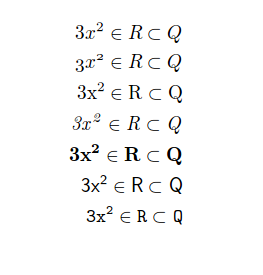In mathematical mode as well as in text mode, you can change the typeface as needed. For instance, it's customary to represent real numbers with a blackboard bold font, or topological spaces with calligraphic font. This article shows several fonts for use in math mode.

# Introduction

For some elements is convenient to have the possibility of changing the font typeface.

Let $$\mathcal{T}$$ be a topological space, a basis is defined as
$\mathcal{B} = \{B_{\alpha} \in \mathcal{T}\, |\, U = \bigcup B_{\alpha} \forall U \in \mathcal{T} \}$Several fonts require the addition of the line \usepackage{amssymb} to the preamble to work.

# Capital letters-only font typefaces

There are some font typefaces that support only a limited number of characters; these fonts usually denote some special sets.

\begin{align*}
RQSZ \\
\mathcal{RQSZ} \\
\mathfrak{RQSZ} \\
\mathbb{RQSZ}
\end{align*}This example shows Calligraphic, Fraktur and Blackboard bold typefaces. For instance, to display the R in blackboard bold typeface $\mathbb{R}$ will do the trick.

# Other mathematical fonts

It's possible to set a different font family for a complete mathematical expression.

\begin{align*}
3x^2 \in R \subset Q \\
\mathnormal{3x^2 \in R \subset Q} \\
\mathrm{3x^2 \in R \subset Q} \\
\mathit{3x^2 \in R \subset Q} \\
\mathbf{3x^2 \in R \subset Q} \\
\mathsf{3x^2 \in R \subset Q} \\
\mathtt{3x^2 \in R \subset Q}
\end{align*}In this case, not only letters but all characters change its appearance, for example $\mathit{3x^2}$ italicises the entire expression.